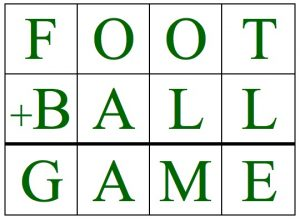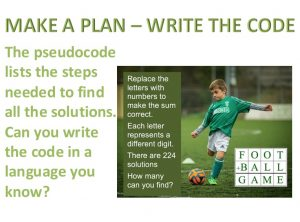Replace the letters in FOOT + BALL = GAME with numbers to make the sum correct. Each letter represents a different digit.

Here is one example: F=1, O=9, T=4, B=6, A=5, L=3, G=8, M=2, E=7

How many solutions can you find?

There are 224 solutions altogether.

You can work with pencil and paper or you might want to use scrap card and make 20 cards, 2 for each digit, all the same size. Then you can try to find solutions by re-arranging 12 of the cards in a 3 by 4 array. Work systematically so you don’t keep trying the same arrangement.

Why not do this as a family group or class investigation? Put a chart on the wall and write up the new solutions as learners find them?

 Examples: F=2, O=9, T=7, B=3, A=4, L=1, G=6, M=0, E=8 2997 3411 + 6408 Replace B,A,L,L here: 2004 BALL + 5981 Replace T, L and E here: 199T 653L + 852E Now find some solutions of your own.The FOOTBALL CHALLENGE Inclusion and Home Learning Guide offers related learning activities on the theme of  Working Systematically suitable for learners of all abilities and all ages from 5 to 18+.